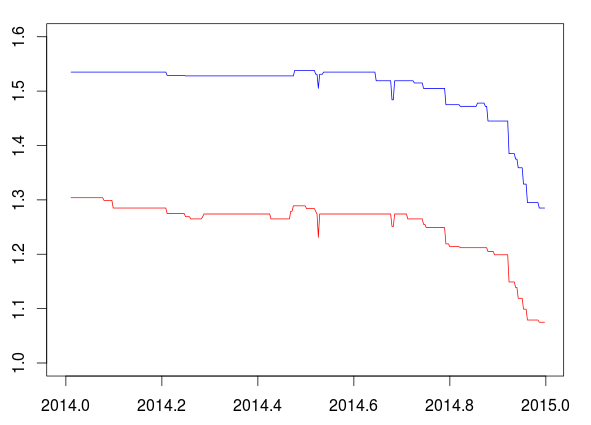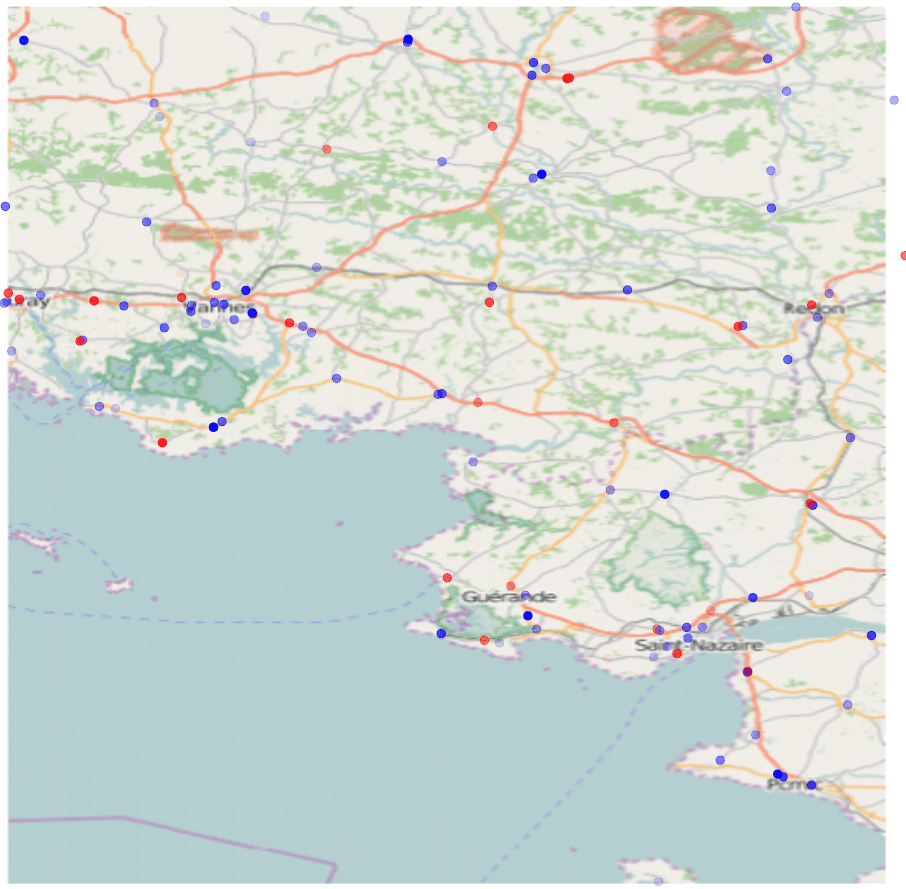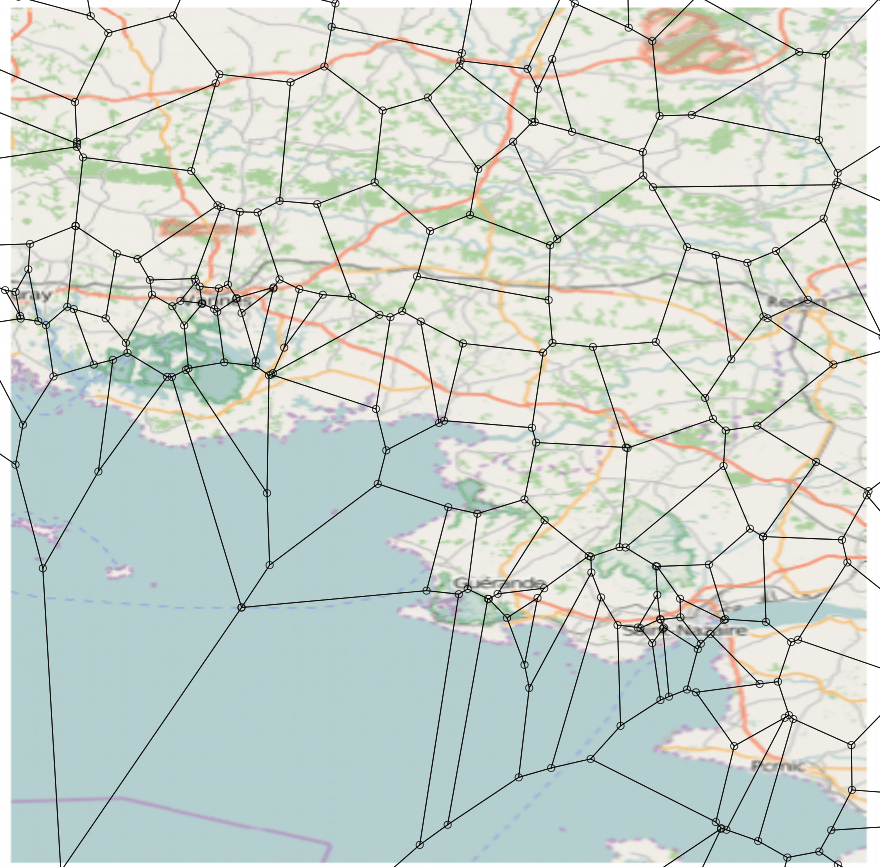Want to share your content on R-bloggers? click here if you have a blog, or here if you don't.

A great think in France, is that we can play with a great database with gas price, in all gas stations, almost eveyday. The file is rather big, so let’s make sure we have enough memory to run our codes,

`> rm(list=ls())`

To extract the data, first, we should extract the xml file, and then convert it in a more common R object (say a list)

```> year=2014
> loc=paste("http://donnees.roulez-eco.fr/opendata/annee/",year,sep="")

Content type 'application/zip' length 15248088 bytes (14.5 MB)

> unzip("oil.zip", exdir="./")
> fichier=paste("PrixCarburants_annuel_",year,
".xml",sep="")
> library(plyr)
> library(XML)
> library(lubridate)
> l=xmlToList(fichier)```

We have a large dataset, with prices, for various types of gaz, for almost any gas station in France, almost every day, in 2014. It is a 1.4Gb list, with 11,064 elements (each of them being a gas station)

```> length(l)
 11064```

There are two ways to look at the data. A first idea is to consider a gas station, and to extract the time series.

```> time_series=function(no,type_gas="Gazole"){
+   prix=list()
+   date=list()
+   nom=list()
+   j=0
+   for(i in 1:length(l[[no]])){
+     v=names(l[[no]])
+     if(!is.null(v[i])){
+       if(v[i]=="prix"){
+         j=j+1
+  date[[j]]=as.character(l[[no]][[i]]["maj"])
+  prix[[j]]=as.character(l[[no]][[i]]["valeur"])
+  nom[[j]]=as.character(l[[no]][[i]]["nom"])
+       }}
+   }
+   id=which(unlist(nom)==type_gas)
+   n=length(id)
+   jour=function(j) as.Date(substr(date[[id[j]]],1,10),"%Y-%m-%d")
+   jour_heure=function(j) as.POSIXct(substr(date[[id[j]]],1,19), format = "%Y-%m-%d %H:%M:%S", tz = "UTC")
+   ext_y=function(j) substr(date[[id[j]]],1,4)
+   ext_m=function(j) substr(date[[id[j]]],6,7)
+   ext_d=function(j) substr(date[[id[j]]],9,10)
+   ext_h=function(j) substr(date[[id[j]]],12,13)
+   ext_mn=function(j) substr(date[[id[j]]],15,16)
+   prix_essence=function(i) as.numeric(prix[[id[i]]])/1000
+   base1=data.frame(indice=no,
+            id=l[[no]]\$.attrs["id"],
+            ville=l[[no]]\$ville,
+  lat=as.numeric(l[[no]]\$.attrs["latitude"])
/100000,
+  lon=as.numeric(l[[no]]\$.attrs["longitude"])
/100000,
+       cp=l[[no]]\$.attrs["cp"],
+       saufjour=l[[no]]\$ouverture["saufjour"],
+       Y=unlist(lapply(1:n,ext_y)),
+       M=unlist(lapply(1:n,ext_m)),
+       D=unlist(lapply(1:n,ext_d)),
+       H=unlist(lapply(1:n,ext_h)),
+       MN=unlist(lapply(1:n,ext_mn)),
+    prix=unlist(lapply(1:n,prix_essence)))
+
+   base1=base1[!is.na(base1\$prix),]
+
+   date_d=paste(year,"-01-01 12:00:00",sep="")
+   date_f=paste(year,"-12-31 12:00:00",sep="")
+   vecteur_date=seq(as.POSIXct(date_d, format =
+                 "%Y-%m-%d %H:%M:%S"),
+                    as.POSIXct(date_f, format =
+                 "%Y-%m-%d %H:%M:%S"),by="days")
+   date=paste(base1\$Y,"-",base1\$M,"-",base1\$D,
+   " ",base1\$H,":",base1\$MN,":00",sep="")
+   date_base=as.POSIXct(date, format =
+                "%Y-%m-%d %H:%M:%S", tz = "UTC")
+   idx=function(t) sum(vecteur_date[t]>=date_base)
+   vect_idx=Vectorize(idx)(1:length(vecteur_date))
+   P=c(NA,base1\$prix)
+   base2=ts(P[1+vect_idx],
+         start=year,frequency=365)
+   list(base=base1,
+        ts=base2)
+ }```

To get the time series, extrapolation is necessary, since we have here observation at irregular dates. Here, for instance, for the second gas station, we get

```> plot(time_series(2)\$ts,ylim=c(1,1.6),col="red")
> lines(time_series(2,"SP98")\$ts,col="blue")```An alternative is to study gas price from a spatial perspective. Given a date, we want the price in all stations. As previously, we keep the last price observed, in each station,

```> spatial=function(dt){
+   base=NULL
+   for(no in 1:length(l)){
+     prix=list()
+     date=list()
+     j=0
+     for(i in 1:length(l[[no]])){
+     v=names(l[[no]])
+     if(!is.null(v[i])){
+       if(v[i]=="prix"){
+   j=j+1
+   date[[j]]=as.character(l[[no]][[i]]["maj"])
+       }}
+   }
+   n=j
+   D=as.Date(substr(unlist(date),1,10),"%Y-%m-%d")
+   k=which(D==D[which.max(D[D<=dt])])
+ if(length(k)>0){
+   B=Vectorize(function(i) l[[no]][[k[i]]])(1:length(k))
+ if("nom" %in%  rownames(B)){
+   k=which(B["nom",]=="Gazole")
+   prix=as.numeric(B["valeur",k])/1000
+   if(length(prix)==0) prix=NA
+   base1=data.frame(indice=no,
+   lat=as.numeric(l[[no]]\$.attrs["latitude"])
/100000,
+   lon=as.numeric(l[[no]]\$.attrs["longitude"])
/100000,
+   gaz=prix)
+   base=rbind(base,base1)
+ }}}
+ return(base)}```

For instance, for the 5th of May, 2014, we get the following dataset

```> B=spatial(as.Date("2014-05-05"))
```

To visualize prices, consider only mainland France (excluding islands in the Pacific, or close to the Caribeans)

```> idx=which((B\$lon>(-10))&(B\$lon<20)&
+ (B\$lat>35)&(B\$lat<55))
> B=B[idx,]
> Q=quantile(B\$gaz,seq(0,1,by=.01),na.rm=TRUE)
> Q=0
> x=as.numeric(cut(B\$gaz,breaks=unique(Q)))
> CL=c(rgb(0,0,1,seq(1,0,by=-.025)),
+ rgb(1,0,0,seq(0,1,by=.025)))
> plot(B\$lon,B\$lat,pch=19,col=CL[x])```Red dots are the most expensive gas stations, that particular day.

If we add contours of the French regions, we get

```> library(maps)
> map("france")
> points(B\$lon,B\$lat,pch=19,col=CL[x])```We can also focus on some specific region, say the South of Brittany.

```> library(OpenStreetMap)
> map <- openmap(c(lat= 48,   lon= -3),
+                c(lat= 47,   lon= -2))
> map <- openproj(map)
> plot(map)
> points(B\$lon,B\$lat,pch=19,col=CL[x])
```As we can see on that map, there are regions that are rather empty, where the closest gas station might be a bit far away. Actually, it is possible to add Voronoi sets on the map, which could help to get the price of the closest gaz station.

```> library(tripack)
> V <- voronoi.mosaic(dB\$lon[id],dB\$lat[id])It is possible to plot each polygon with the color of the gaz station we add. Actually, it is a bit tricky, and I could not find a R function to to this. So I did it manually,

```> plot(map)
> P <- voronoi.polygons(V)
> library(sp)
> point_in_i=function(i,point) point.in.polygon(point,point,P[[i]][,1],P[[i]][,2])
> which_point=function(i) which(Vectorize(function(j) point_in_i(i,c(dB\$lon[id[j]],dB\$lat[id[j]])))(1:length(id))>0)
> for(i in 1:length(P)) polygon(P[[i]],col=CL[x[id[which_point(i)]]],border=NA)```With this map, we can see that we have blue areas, i.e. all stations in a given area are cheap (because of competition), but in some places, a very expensive one is next to a very cheap one. I guess we should look closer at the dynamics… [to be continued….]